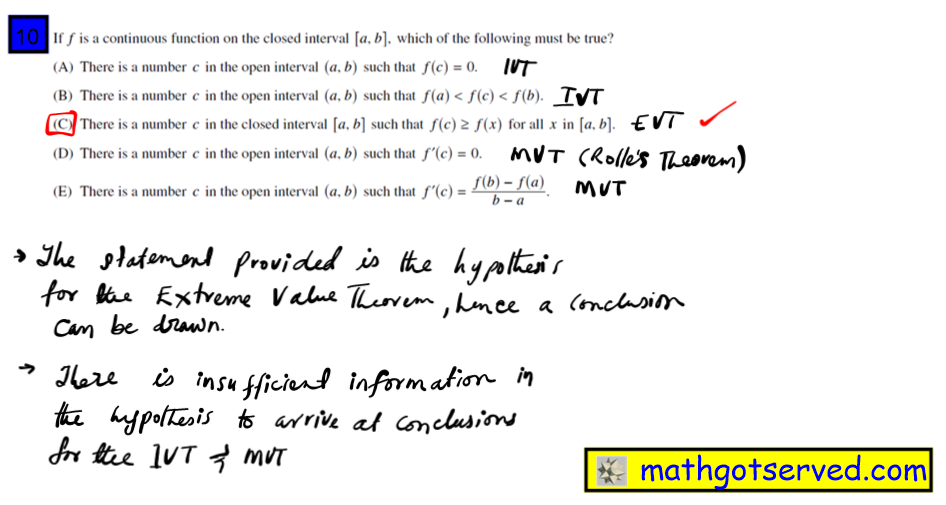MATHGOTSERVED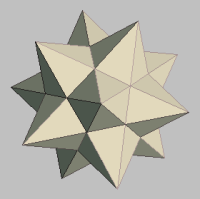LIMITS AND CONTINUITY
LIMITS
AP EXAM REVIEW
LIMITS
DERIVATIVES
APP DERIVATIVES

INTEGRALS

APP INTEGRALS
DIFF. EQUATIONS

# Case 1 Point: When approaching a point defined or not (Closed or Open) the limit is the y coordinate of the point you are approaching.  Case 2 Vertical Asymptote: When approaching a vertical asymptote, the limit is infinity if you are heading up and negative infinity if you are heading downwards. Case 3 Oscillation: When a function oscillates toward a boundary x  value the limit in that case does not exist.

INTERACTIVE PRACTICE TEST
FORMULA SHEET & FLAHS CARDS

# STRATEGY FOR FINDING FINITE DIRECTIONAL LIMITS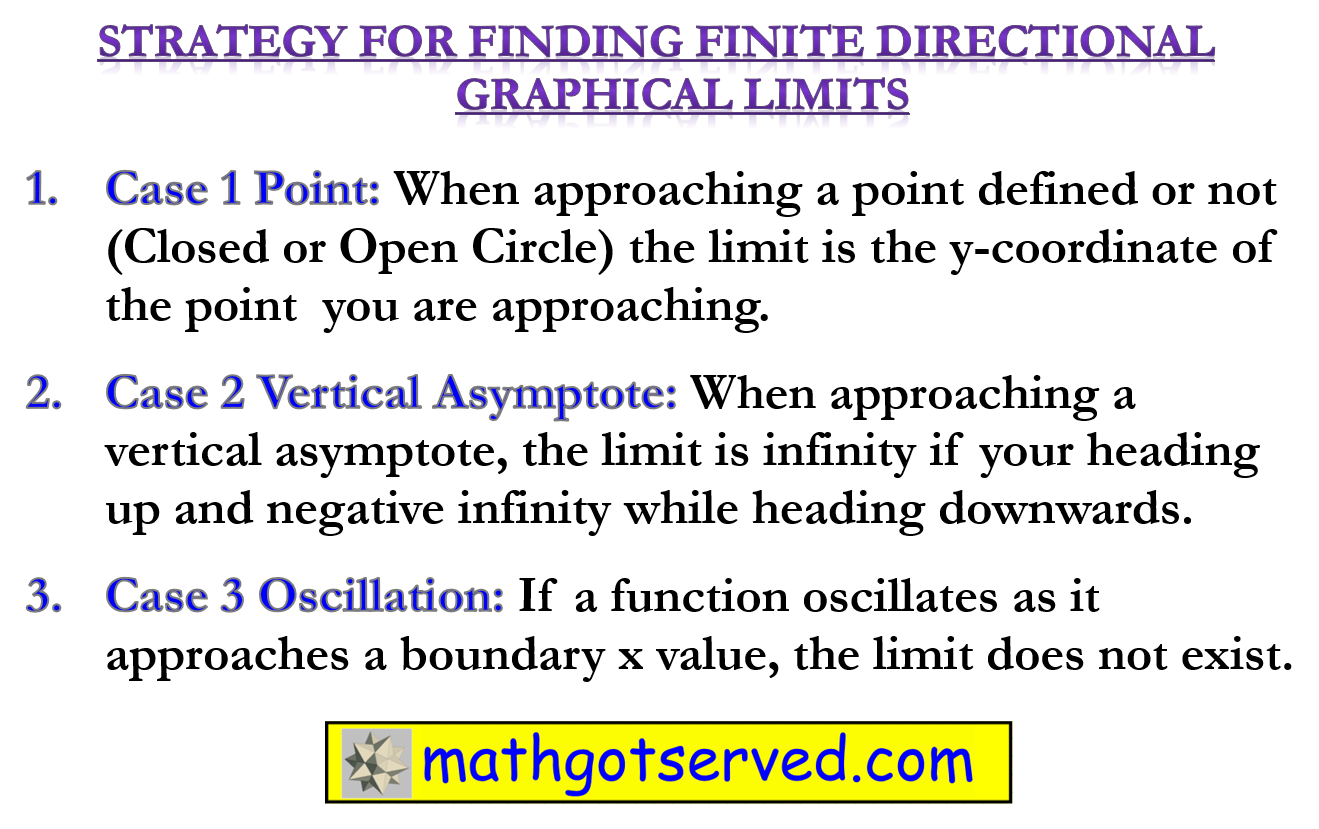# Ap Calc Limits Practice Test 2

Please wait for the Test to Load.....
How to (Procedures) for Finding Finite Limits Algebraically (steps)
1.Establish the fact that the limit is finite. (i.e The value that x and the f(x) approaches must not be ±∞)
2.Substitute the value x is approaching and evaluate. If 0 is in the denominator go to 3.
3.Factor, or rationalize the numerator or denominator and cancel out any removable discontinuities and substitute again. If 0 at the bottom use a non algebraic approach since the limit might not exist.
Procedure for Finding Graphical Limits
Point: Find the y coordinate of the point that you are approaching from that direction.
Horizontal Asymptote: Find the y coordinate of the equation of the line
Vertical Asymptote: One sided +/- infinity, Double sided  does note exist.
The limit of a function is the value the function approaches as the independent variable approaches a value, negative or positive infinity.
“Read as the limit of f(x) as x approaches a is L”
One Sided Limit
Right/Left Hand Limit: The right/left hand limit of a function f(x) as x approaches a equals L is written as:
One Sided LimitIt means that if we get take x to be sufficiently close to the right/left of a then f(x) the value of the function will be arbitrarily close to L.
Chapter 1 Prerequisites of Calculus
Chapter 2: Limits and Continuity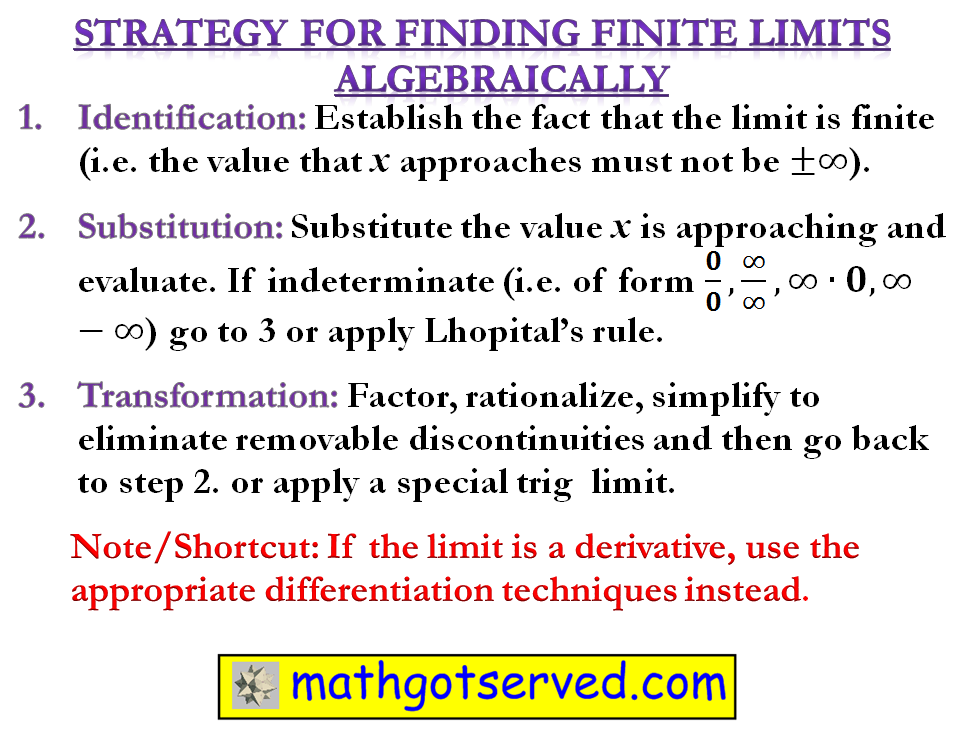# Why Calculus? A Preview

1.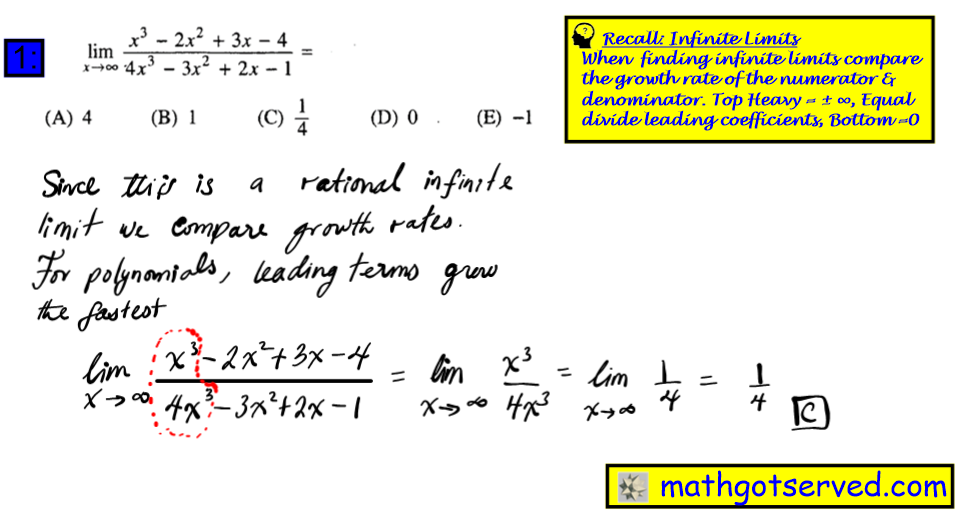2.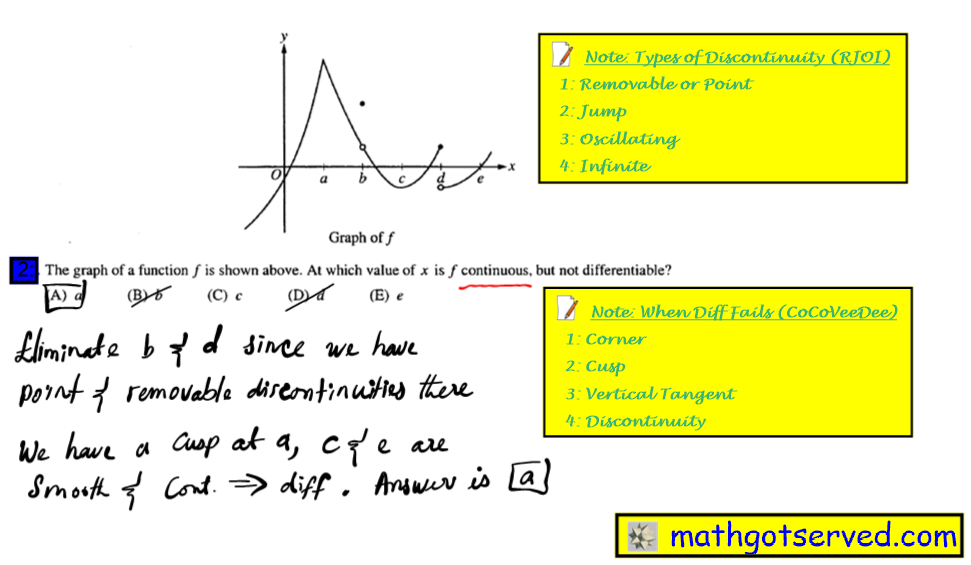3.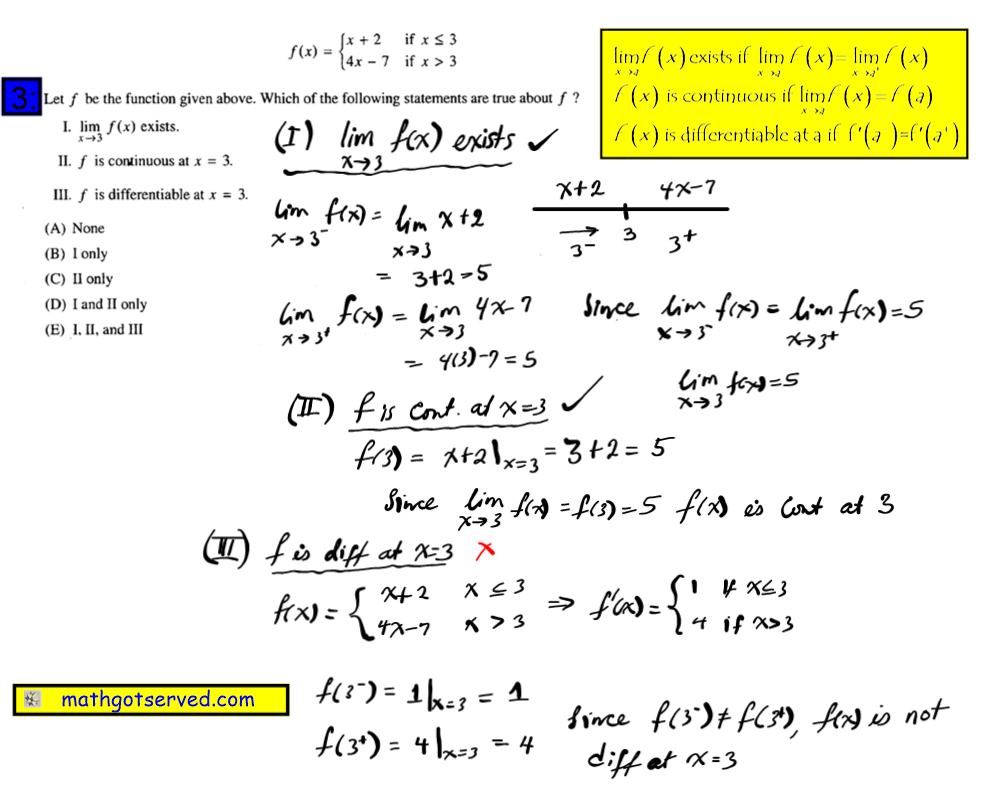4.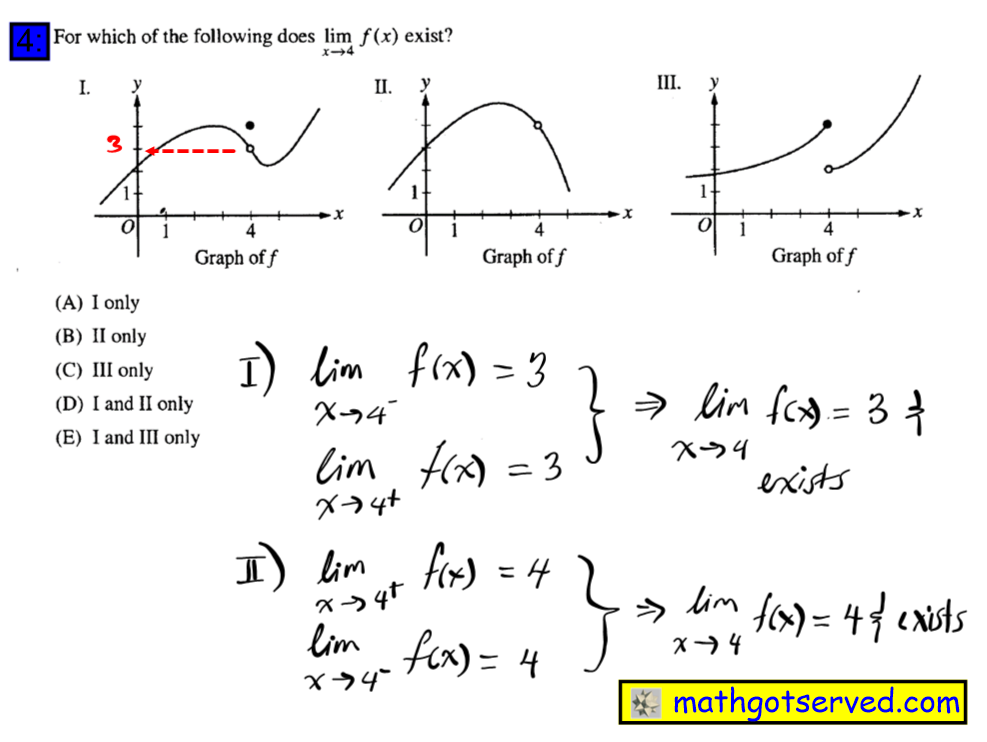5.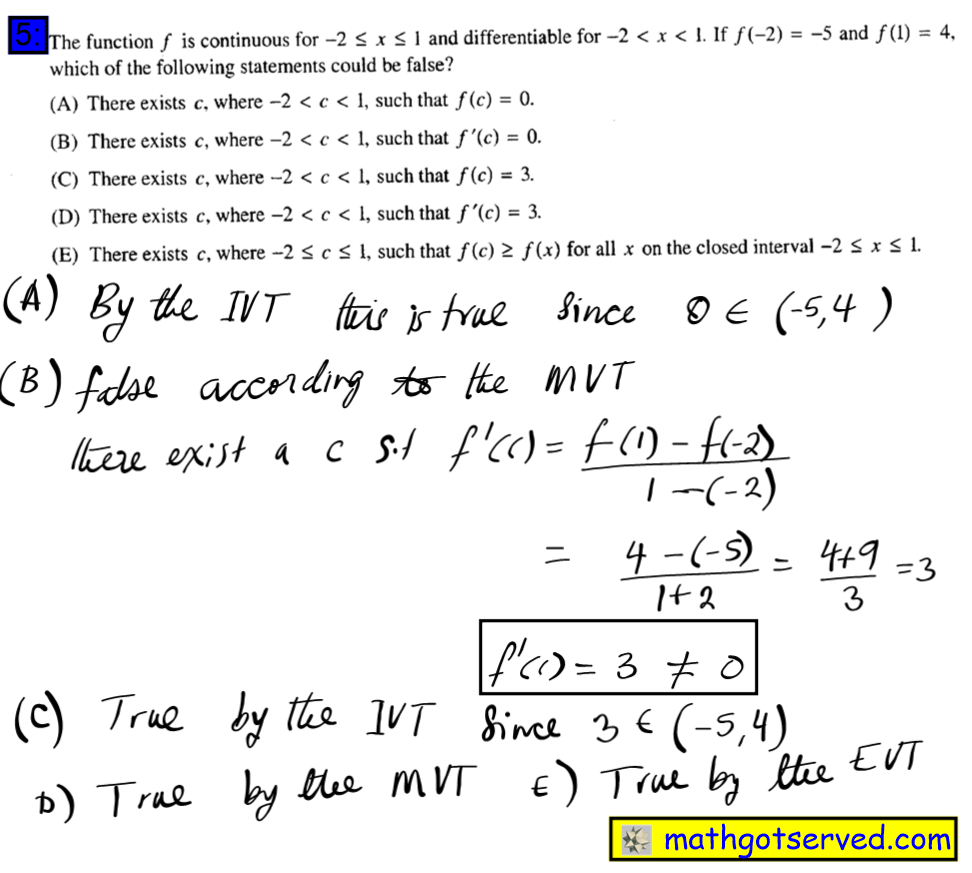6.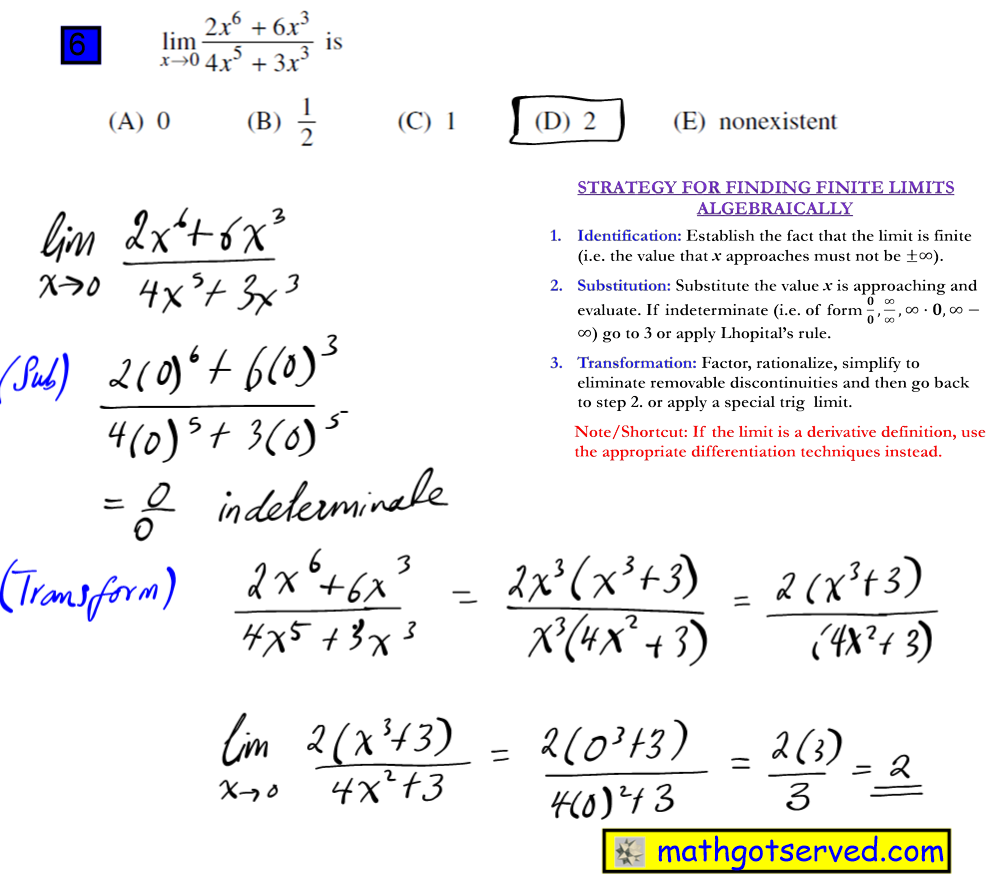7.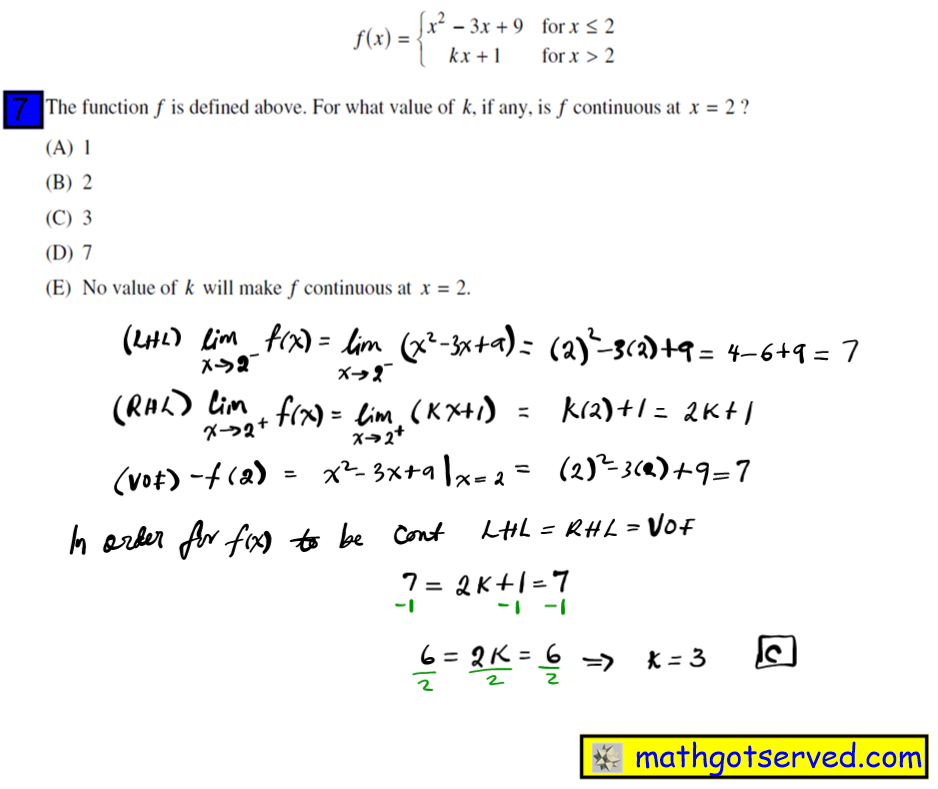8.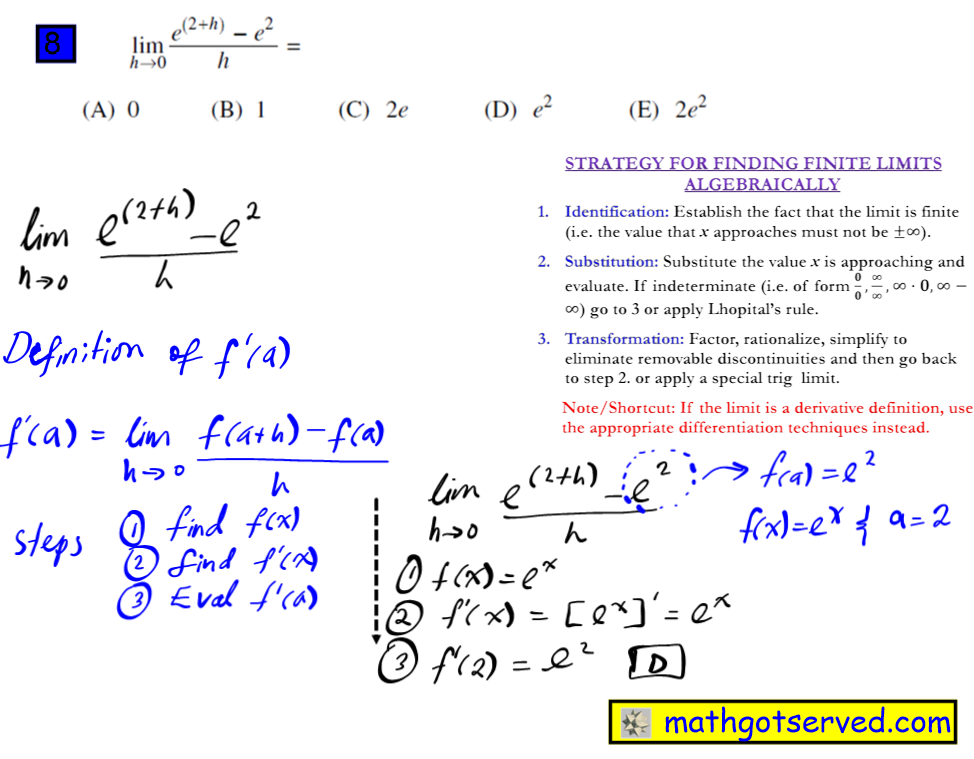9.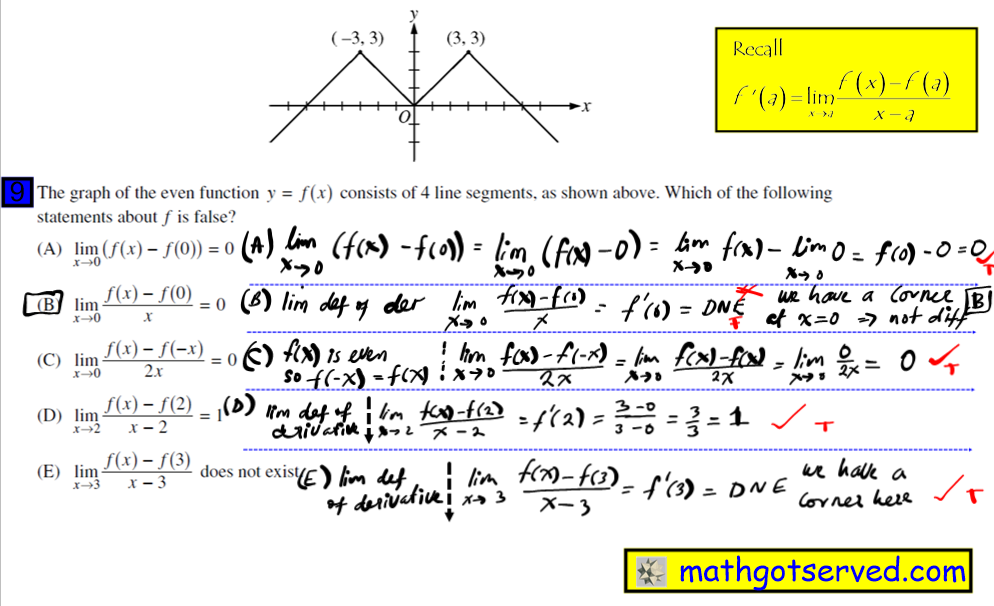10.